# Redox ReactionsPage 1

#### WATCH ALL SLIDES

Slide 1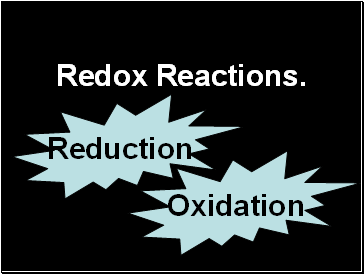## Redox Reactions.

Oxidation

Reduction

Slide 2## GCSE

Oxidation:

Gain of oxygen

Loss of electrons

Reduction:

Loss of oxygen

Gain of electrons

Increase in

oxidation

number

Decrease in

oxidation

number

Slide 3## 4 Experiments:

Burning magnesium

Copper in silver nitrate solution

Chlorine solution and potassium iodide solution

Exploding hydrogen

Word equation

Balanced symbol equation

Slide 4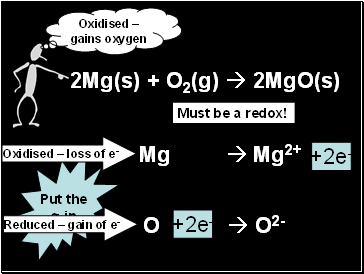2Mg(s) + O2(g)  2MgO(s)

Oxidised – gains oxygen

Must be a redox!

Mg  Mg2+

O  O2-

Put the

e- in.

+2e-

+2e-

Oxidised – loss of e-

Reduced – gain of e-

Slide 5Cu(s) + 2AgNO3(aq)  Cu(NO3 )2(aq) + 2Ag(s)

Ag+  Ag

Cu  Cu2+

Complete the half-equations

+e-

+2e-

Oxidised?

Reduced?

Oxidised – loss of e-

Reduced – gain of e-

Slide 6Try Question 1.

Slide 7H2(g) + ½ O2(g)  H2O(g)

Covalent!

No H+ or OH-

Need a new

definition.

Slide 8GCSE

Oxidation:

Gain of oxygen

Loss of electrons

Reduction:

Loss of oxygen

Gain of electrons

Increase in

oxidation

number

Decrease in

oxidation

number

Slide 9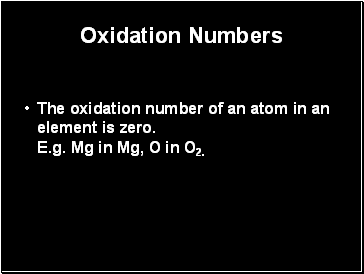## Oxidation Numbers

The oxidation number of an atom in an element is zero. E.g. Mg in Mg, O in O2.

Slide 10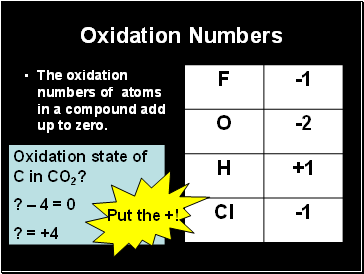Oxidation Numbers

The oxidation numbers of atoms in a compound add up to zero.

Oxidation state of C in CO2?

? – 4 = 0

? = +4

Put the +!

Slide 11Oxidation Numbers

The oxidation numbers of atoms in a compound add up to zero.

Oxidation state of Mg in MgCl2?

+2

Slide 12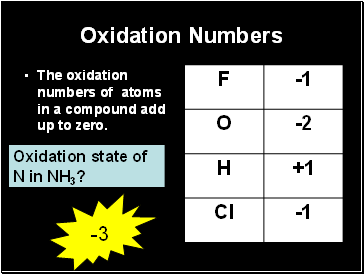Oxidation Numbers

The oxidation numbers of atoms in a compound add up to zero.

Oxidation state of N in NH3?

-3

Slide 13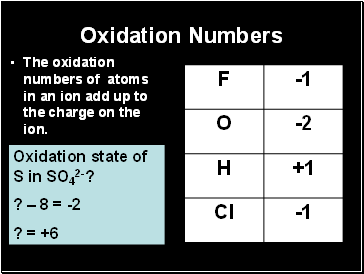Go to page:
1  2